﻿ Estimation of Short and Long Run Equilibrium Coefficients in Error Correction Model: An Empirical Evidence from Nepal

### Estimation of Short and Long Run Equilibrium Coefficients in Error Correction Model: An Empirical Ev...

Kamal Raj DhungelOPEN ACCESSPEER-REVIEWED

## Estimation of Short and Long Run Equilibrium Coefficients in Error Correction Model: An Empirical Evidence from Nepal

Kamal Raj DhungelCentral Department of Economics, Tribhuvan Univercity, Kathmandu, Nepal

### Abstract

This study aims to investigate the short and long run equilibrium between the electricity consumption and foreign aid of Nepalese economy during 1974-2012. Unit root test, co-integration test and finally error correction model are the econometric tools to establish the relationship between electricity consumption and foreign aid. In addition to this ordinary least square method is used to find out the foreign aid elasticity and spurious regression. The findings reveal that the variables are non-stationary at their level and they become stationary in their first difference. There are two co-integration equations showing the long run relationship between electricity consumption and foreign aid. There is short and long run equilibrium as indicated by the statistically significant coefficient of foreign aid and error correction term. The long run elasticity coefficient reveals that the 1% change in foreign aid will change the electricity consumption by 0.46%. The results of ECM indicate that there is both short and long run equilibrium in the system. The coefficient of one period lag residual is negative and significant which represent the long run equilibrium. The coefficient is -0.336 meaning that system corrects its previous period disequilibrium at a speed of 33.6% annually to reach at the steady state.

### At a glance: Figures

1
Prev Next

• Dhungel, Kamal Raj. "Estimation of Short and Long Run Equilibrium Coefficients in Error Correction Model: An Empirical Evidence from Nepal." International Journal of Econometrics and Financial Management 2.6 (2014): 214-219.
• Dhungel, K. R. (2014). Estimation of Short and Long Run Equilibrium Coefficients in Error Correction Model: An Empirical Evidence from Nepal. International Journal of Econometrics and Financial Management, 2(6), 214-219.
• Dhungel, Kamal Raj. "Estimation of Short and Long Run Equilibrium Coefficients in Error Correction Model: An Empirical Evidence from Nepal." International Journal of Econometrics and Financial Management 2, no. 6 (2014): 214-219.

 Import into BibTeX Import into EndNote Import into RefMan Import into RefWorks

### 1. Introduction

Hydropower is a promising sector if developed rationally can transform Nepal into prosperity. Having more than a century long hydropower development history Nepal has a disappointed progress. Nearly 1.5% of the potential capacity of more than 42 thousand MW is being harnessed. Most of the existing hydropower projects (HP) from the beginning to date are constructed through foreign assistance. Beginning, up to the period of 1980, was with grants primarily assisted by Britain, India, China and Russia. Some other built from the multilateral aid most of which in the form of loan. A few with small capacities are built through foreign direct investment. In recent days some HP are built from the domestic resource mobilization. But the scope of domestic resource mobilization is limited which implies that Hydropower seeks foreign capital to transform hydropower potentiality into reality. It proves that Nepal is becoming an aid dependent country. It is because of its limited and unmanaged internal resources to invest in socio-economic development. Infrastructure projects require huge investments that the government is incapable of. “Successful development requires public investments, but governments in impoverished countries are often too cash strapped and too indebted to finance the requisite investments. When the government is unable to build the roads, a power grid and other basic infrastructure the private sector languishes the result in a fiscal policy trap in which poverty leads to low public investments and low public investments reinforce poverty. This kind of fiscal collapse is one of the most important causes of economic development failures in the poorest countries” (Sachs, 2008 p.223). In Nepal, the private sector is reluctant to invest in infrastructure because of the long gestation period bound by the risk of political instability. In spite of this, private sector has been involving in generating electricity from its immense water resource. But the rate of investment in this sector is not encouraging. It implies that existing hydropower projects keeping constant a few in exception were built either from foreign loan or from grant. In this light, aid played vital role in the development of hydropower projects. Keeping a few cases given and constant, all the hydropower projects small or big have largely been influenced by foreign aid. At the beginning, aid in the form of grants played an important role in construction of hydropower projects. But foreign assistance in the form of grants has been changing over time. Grant at large is being replaced by loans as bilateral donors are gradually changing into multilateral. The role of foreign aid, be it in the form of grant or loan in harnessing hydropower is a hot button issue. In this context this article aims to answer the question like is there short and long run equilibrium between electricity consumption and foreign aid?

### 2. A Brief Survey of Previous Work

A number of studies have been conducted to investigate the casual relationships between energy consumption and economic growth but with few studies about foreign aid. Aqueel and Butt (2001) studied the causal relationship between energy consumption and economic growth. To investigate the causal relationship among the stated variables, they prefer to use the integration and Granger tests. They found unidirectional causality running from economic growth to petroleum consumption and causality running from economic growth to gas consumption. Dhungel (2014a) has applied vector error correction model to determine the short and long run causality between the variable gross domestic product and remittance. Evidence has not support the hypothesis of remittance causes gross domestic product in the long run but there is strong evidence about the short run causality running from remittance to gross domestic product. But opposite is true in reverse order. Gross domestic product causes remittance in both short and long run. Mozumdar and Marathe have applied vector error correction model (VECM) to explore the dynamic Granger causality. They found that per capita gross domestic product Granger causes per capita energy consumption. Dhungel (2008) has found a unidirectional running from coal, oil and commercial energy to per capita real GDP and a unidirectional causality from per capita real GDP to per capita electricity consumption. Mashih and Mashih (1996) consider six Asian economies to examine the temporal causality between energy consumption and income. They have applied vector error correction model (VECM). Their finding shows that energy consumption is causing income in India, income is causing energy consumption in Indonesia, bi-directional causality exists in Pakistan. However, they use an ordinary vector autoregressive (VAR) model for the rest three countries (Malaysia, Singapore and the Philippines). Their investigation failed to find any causality between energy consumption and income. Dhungel (2009) has investigated, causal relationship between the per capita electricity consumption and GDP during the period 1980-2006 in Nepal using co-integration and vector error correction model. He found unidirectional causality from per capita real GDP to per capita electricity consumption and but not otherwise. Zaman K et al.(2012) have found that determinants of electricity consumption function are co-integrated and influx of foreign direct investment, income and population growth is positively related to electricity consumption in Pakistan. However, the intensity of these determinants was different on electricity consumption. If there is 1% increase in income, foreign direct investment and population growth; electricity consumption increases by 0.973%; 0.056% and 1.605% respectively.

Dhungel (2014c) has applied error correction model to investigate the short and long run equilibrium between the variables electricity consumption as dependent variable and foreign and GDP as explanatory variables during the period 1974-2011. The results of ECM indicate that there is both short- and long-run equilibrium in the system. The coefficient of one period lag residual coefficient is negative and significant which represents the long-run equilibrium. The coefficient is -0.72 meaning that system corrects its previous period disequilibrium at a speed of 72% annually. Dhungel (2014b) has applied error correction model to investigate the equilibrium position between electricity consumption and foreign aid during the period 1974-2011. He found that disequilibrium will be restored by the speed of 34% annually to reach the economy in the steady state.

### 3. Estimation Method

3.1. Variables and Data Sources

Electricity consumption (EC) in million KWh over the period 1974-2012 is the dependent variable. Foreign aid (FA) in million rupees comprising loan and grant over the same period of time is the explanatory variable. The data of these variables are collected from the ministry of i) finance, ii) energy, Central bureau of statistics, Nepal Rastra Bank and other published sources. First of all the collected data of all the variable under consideration has been converted into per capita terms to capture the effect of population growth and converting them into natural logarithm.

3.2. Model Specification

Generally time series data are non-stationary if used to run regression may produce spurious regression which is not desirable. Saying the same thing again, regression of a non-stationary time series on another non-stationary time series may cause a spurious regression. Running regression on the non-stationary series at their level would generally be produced spurious regression. In such a case Durbin-Watson (DW) statistics may be less than the value of R-squared. If R-squared value is found greater than DW statistic, it definitely implies the symptom of the spurious regression. But instead, the residual of the model is found stationary, the model under consideration would not be no longer spurious regression. Therefore, OLS estimation of the given non-stationary time series data is a necessary condition for the estimation of R-squared, DW statistic and residual (error term) which are used to detect spurious regression. If the model is non-spurious then the variables in the model are co-integrated or they have long run relationship or equilibrium relationship between them. Then it is a long run model and estimated coefficients are long run coefficients. The model is given by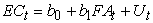(1)

Where, EC = Electricity consumption in million KWh,

FA = Foreign aid in million rupees

U = Error term (residual-difference between observed and estimated values)

t = time

b0, (intercept) b1(slop coefficient) are parameters to be estimated and they represent long run coefficients.

3.2.1. Unit Root Test

Generally, time series data contains unit root meaning that these series are not stationary. Augmented Dickey Fuller (ADF) test (1979), generally popular method, is being applied to test the unit root under the hypothesis series has unit root.Akaike criterion has been followed to lag selection. The model to check the unit root is: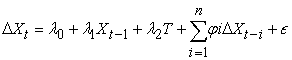(2)

Where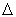is the difference operator X is the natural logarithm of the series. T is a trend variable. λ and ψ are parameters to be estimated and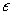is the error term.

3.2.2. Johansen Co-integration Test

Johansen co-integration test procedure consists of estimating a vector autoregressive (VAR) models which includes difference as well as the levels of the non-stationary variables. The equation for Johansen co-integration test is given by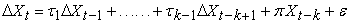(3)

Where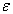is Gaussian random variable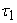and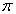are matrices of parameters estimated using OLS. The component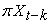produces different linear combinations of levels of the time series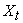as such the matrixcontains information about the long run properties of the system describe by the model. For instance, if the rank of the matrixis 0, then no series of the variables can be expressed as a linear combination of the remaining series. This indicates that there does not exists a long run relationship among the series of the VAR model as a test of co-integration a rank of 0 means integration is rejected. On the other hand, if the rank of the coefficient matrixis 1, or greater than 1 then there exists 1 or more co-integrating vectors. This indicates a long run relationship or that the series exhibits significant evidence or behaving as a co-integrated system. Johansen (1988) and Engle and Grnger (1987) proposed two statistics which can be used to evaluate the rank of the coefficient matrix or the number of co-integrating vectors. The one used here is the a likelihood ratio test of the null hypothesis that the number of is r versus the alternative r+1 vectors. In this case Ho is that the number of co-integrating vectors equals 0.

3.2.3. Error Correction Model

Finally, short and long run equilibrium has been investigated with the help of error correction model (ECM) which is an appropriate system of single equation. The error correction model tells us the degree to which the equilibrium behavior drives short run dynamics. Equilibrium relationship in turn have implications for a short run behavior, one or more series move to restore equilibrium. Government support falls with poor economic conditions and improves in the economic booms, adversarial behavior is matched by increasingly confrontational responses increase in spending decreases in liberal policy preferences and period of sustained economic growth produces in party support. In each case, short run change is necessary to maintain the long run relationships (Boef, 2000). Short and long run equilibrium between the variable EC and FA in the system have been investigated with the help of ECM as given below.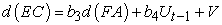(4)

d(EC) = first difference of electricity consumption

d(FA) = first difference of foreign aid

Ut-1 = One period lag of residual obtained from the OLS estimation (equation 1).

b3 and b4 are parameters to be estimated

V = Error term

Parameters b3 irrespective of its sign but should be individually significant represent short run equilibrium between EC and FA. However, parameter b4 represents long run equilibrium between the same variable. The sign of b4 should be negative and significant as well for holding long run equilibrium.

### 4. Empirical Findings

4.1. Graphical Representation of Data

Both variable EC and FA are non-stationary. First set of graphs represent the non-stationary series. In the similar way, second set of graphs represent the stationary series.

4.1.1. Graphs of Non-stationary Series

A graphical view of non-stationary series is given in Figure 1. The graph of all the two variables indicated by EC and FA are non-stationary.

4.1.2. Graphs of Stationary Series

Figure 2 is a graphical view of stationary series. Presented graph of all the series indicated by DEC and DFA are being drawn after the corresponding data has been converted into first difference.

4.1.3. Spurious Regression
4.1.4. OLS Estimation Results at Level

The purpose of OLS estimation in level is to detect the spurious regression. If the results found spurious, they will not be able to further processing or use. Such results if used to apply wrong things will guide to formulate policies in the economy. The estimated result shows that R-square is greater than the DW statistics which is the fundamental criteria for having spurious regression. The results of these statistics estimated using equation (1) are given in Table 1.

#### Table 1. R-square and DW Statistics

These results (Table 1) clearly prove that the OLS estimation is seriously affected by the spurious regression as the estimated coefficient R-square (0.89) is greater than the estimated coefficient of DW Statistics (0.84). The estimated coefficients from such regression cannot be called best estimation. Moving average and LM test are being applied in order to correct the OLS estimation. The results are given in Table 2.

#### Table 2. Results of OLS parameter estimation at level

Table 2 represents results from the ordinary least square estimation of the relationship between EC and FA. F-statistic (205.5 with probability 0) shows that over all estimation is significant at 1% level and has a strong explanatory power (R-squared is 0.92). Individual coefficients are also significant at 1% level as indicated by t-statistic. Both LM test and DW test shows that the estimation is not affected by the serial correlation. It appears from these results that electricity consumption and foreign aid are positively correlated over the time period of 1974-2012. The growth elasticity of foreign aid in that time period is 0.46. It indicates that the 1% change in foreign aid will change the electricity consumption by 0.46%. The elasticity coefficient of FA is less than 1 indicating a less proportional change in electricity demand associated with the change in FA. From this finding it can be inferred that aid coming from abroad has not been used in productive sector that helps to achieve high economic growth rate. It is because with the increase in aid has not helped to increase economic growth that in turn helped to increase electricity consumption.

### 5. Unit Root Test

5.1. Observed Variable

The finding of the ADF test exhibits that both series EC and FA are non-stationary in their level. However, stationarity is found after first deference. It indicates that they are in the same order that is I(1). It is the fundamental criteria to examine the long run relationship between the variables EC and FA. The appropriate lag order is 3 selected by using Akaike criteria. The ADF test results estimated using equation (2) are given in Table 1.

5.2. Residual

As shown in Table 1, results show that R-squared value is greater than DW statistic value showing a symptom of spurious regression. The corrective measure is that if the residual denoted by U of equation 1 is stationary at level it would be desirable to accept the model for further analysis even at a situation in which R-squared is greater than DW statistic. It is the established alternative criteria for accepting the model if spurious as proved by R-squared and DW statistic. Table 4 shows the stationarity of residual (U) obtained from the estimation of equation 1 at level as shown by ADF test with null hypothesis U has a unit root.

5.3. Co-integration Test

Variables EC and FA are I(1) as indicated by ADF test that allow us to estimate the co-integration test to determine the long run relationship. Table 5 represents the Johansen co-integration test results estimated by using equation (3). The purpose of this equation is to determine the long run relationship or co-movement between the series under consideration. Test results shows that there are two co-integrating equations indicating a long run relationship between variables (EC and FA).

5.4. Error Correction Model (ECM)

Co-integration and non-spurious regression are the fundamental requirements of ECM. Results of co-integration test (Table 5) provide enough evidence on the long run relationship between the variables under consideration as there are two co-integration equations. Result of ADF test provides enough evidence of stationarity of residual (Table 4) at level. Both these two conditions have proved that EC and FA are co-integrated and non-spurious and formed a basis to estimate ECM (equation 4). The results of ECM are given in Table 6.

#### Table 6. Results of OLS parameter estimation in first difference

The ECM is no spurious regression model as indicated by the R-squared and DW statistics. The coefficient b3 is positive indicating there is positive relationship between d(EC) and d(FA). b3 is significant at 5% level as indicated by t-test. This represents the short run equilibrium coefficient. The coefficient b4 is long run equilibrium coefficient which also is known as the error correction coefficient. It is negative and significant as desired (Table 6). b3 and b4 are the coefficients of D(FA) and Ut-1 respectively as shown in equation (4).

### 6. Equilibrium

The primary purpose of this paper is to find out the equilibrium position of the variables (EC and FA). As define in equation (4) b3 and b4, a coefficient of d(FA) and one period lag error correction term (Ut-1) represent the equilibrium position in the short and long run respectively. The estimated values of these parameters are given in Table 7.

#### Table 7. Short and long run equilibrium

6.1. The Short Run Equilibrium

The estimated value of b3 is 0.114. It is individually significant at 5% level (Table 7).This coefficient represents the short run coefficient and represent the short run equilibrium. It tells about the rate at which the previous period disequilibrium of the system is being corrected. The value of b3 is 0.114 meaning that system corrects its previous period disequilibrium at a speed of 11.4% between variables EC and FA.

6.2. The Long Run Equilibrium

Ut-1 is one period lag error correction term or residual. It guides the variables (EC and FA) of the system to restore back to equilibrium or it corrects disequilibrium. To happen this, the sign of this should be negative and significant. Parameter b4 represents its coefficient. It tells about the rate at which it corrects the previous period disequilibrium of the system if it is negative and significant. The coefficient of b4 is negative (-0.336, Table 7) and is significant at 1% level meaning that system corrects its previous period disequilibrium at a speed of 33.6% annually. It implies that the model identified the sizable speed of adjustment by 33.6% of disequilibrium correction yearly for reaching long run equilibrium steady state position.

### 7. Conclusion

A strong relationship exists between electricity consumption and foreign aid over the period of 1974-2012. The regression model is not spurious as tested. The time series data of these variables contain unit root and they become stationary after conducting ADF test. They have long run relation as indicated by Johansen co-integration test. The statistically significant elasticity coefficient of OLS estimation at level expresses that the 1% change in foreign aid will change the electricity consumption by 0.46%. It exhibits that increasing volume of aid has not helped to increase economic growth that in turn helped to increase electricity consumption. Thus, Nepal should formulate policies that can help to mobilize foreign aid in the productive sector in order to achieve desired economic growth that can increase electricity consumption and in turn create gainful employment to the mass of Nepalese people to come. The results of ECM indicate that there is both short and long run equilibrium in the system. The coefficient of one period lag residual coefficient is negative and significant which represent the long run equilibrium. The coefficient is -0.336 meaning that system corrects its previous period disequilibrium at a speed of 33.6% annually.

### References

  Aqeel, A. and M.S. Butt, 2001, “The relationship between energy consumption and economic growth in Pakistan”, Asia Pacific Development Journal 8(2) pp. 101-110.In article  Boef, S. De, 2000, “Modeling equilibrium relationships: Error correction model with strongly autoregressive data”, Pensilvania University, Department of Political Science, pp. 78-94In article  Dhungel, K.R., 2008, “A causal relationship between energy consumption and economic growth in Nepal”, Asia-Pacific Development Journal, 15(1) PP. 137-150.In article  Dhungel, K.R., 2009, ‘Does economic growth in Nepal cause electricity consumption’ Hydro Nepal Issue 5, pp. 47-51In article  Dhungel, K.R., 2014a, “Does Remittance in Nepal Cause Gross Domestic Product? An Empirical Evidence Using Vector Error Correction Model.” International Journal of Econometrics and Financial Management 2(5), pp. 168-174.In article  Dhungel, K.R., 2014b, “Short and Long Run Equilibrium between Electricity Consumption and Foreign Aid”, Review of Contemporary Business Research 3(2), pp. 105-115In article  Dhungel K.R., 2014c, “On the relationship between electricity consumption and selected macroeconomic variables: empirical evidence from Nepal” Modern Economy, 5(4), pp. 360-366。In article CrossRef  Dickey, D. A., and Fuller W. A., 1979, “Distribution of the Estimators for Autoregressive Time Series with a Unit Root”, Journal of the American Statistical Association 74, pp. 427-431In article  Engle R. and Granger C.,1987, “Co-integration and error correction; representation, estimation and testing”, Econometrica, 55, pp-251-276In article  Johansen, Soren, 1988, “Statistical analysis of cointegration vectors,” Journal of Economic Dynamics and Control, Elsevier, vol. 12(2-3), pp. 231-254.In article CrossRef  Mozumder, P. and A. Marathe, “Causality relationship between electricity & GDP in Bangladesh”, Energy Policy (www.unm.edu).In article  Mashih, A.M.M. and R. Mashih, 1996, “Energy consumption real income and temporary causality results from multicountry study based on co-integration and error correction modeling techniques”, Energy Economics 18, pp. 165-83.In article CrossRef  Sachs, J., 2008, “Common Wealth, Economics of Crowded Planet”, Penguin Books.In article  Zaman K., Muhammad M. Khan, Mehboob Ahmad, RabiahRustam, 2012, “Determinants of electricity consumption function in Pakistan: Old wine in a new bottle”, Energy Policy Vol.50, pp. 623-34.In article CrossRef